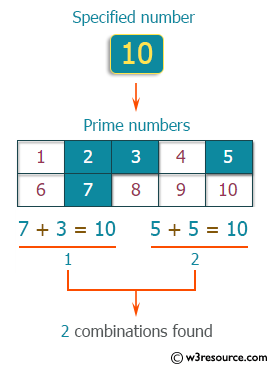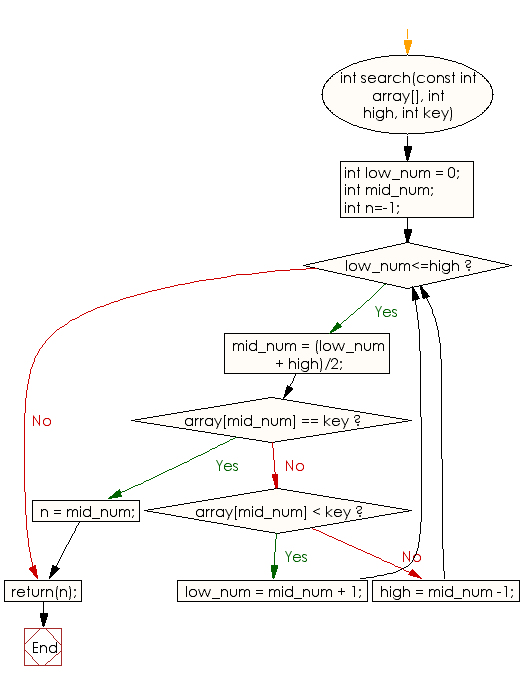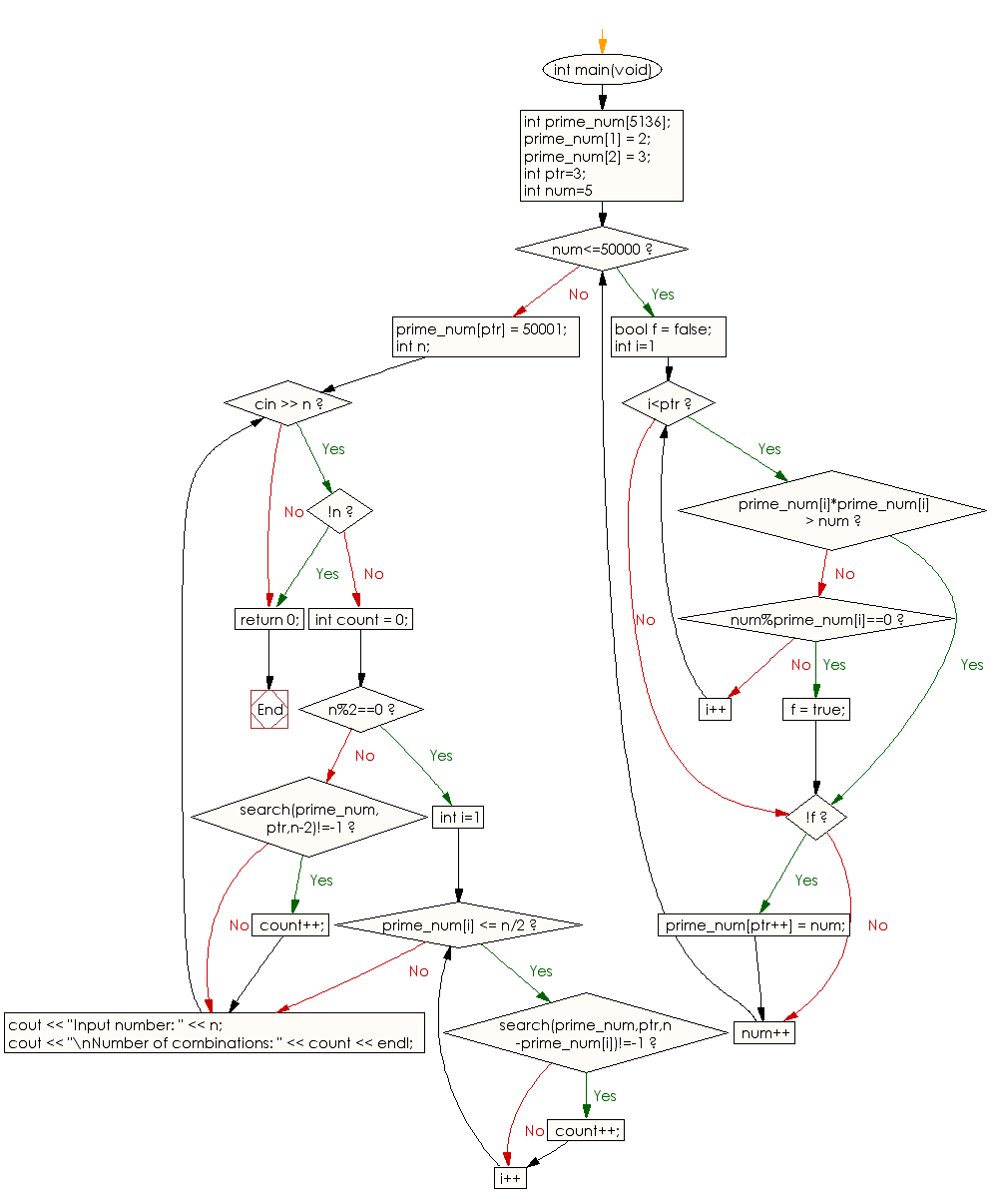﻿ C++ : Combinations that express n as a sum of two prime

# C++ Exercises: Accept an integer (n) from the user and outputs the number of combinations that express n as a sum of two prime numbers

## C++ Basic: Exercise-76 with Solution

An even number of 4 or more can be represented by the sum of two prime numbers. This is called the Goldbach expectation, and it is confirmed that it is correct up to a considerable number by computer calculation. For example, 10 can be expressed as the sum of two prime numbers 7 + 3, 5 + 5. Write a C++ program that accepts an integer (n) from the user and outputs the number of combinations that express n as the sum of two prime numbers.
Note: n should be greater than or equal to 4 and less than or equal to 50,000.

Pictorial Presentation:Sample Solution:

C++ Code :

``````#include <iostream>
using namespace std;

int search(const int array[], int high, int key)
{
int low_num = 0;
int mid_num;
int n=-1;
while(low_num<=high){
mid_num = (low_num + high)/2;
if( array[mid_num] == key ){
n = mid_num;
break;
}else if ( array[mid_num] < key){
low_num = mid_num + 1;
}else{
high = mid_num -1;
}
}
return(n);
}

int main(void){
int prime_num;
prime_num = 2;
prime_num = 3;
int ptr=3;
for(int num=5; num<=50000; num++){
bool f = false;
for(int i=1; i<ptr; i++){
if(prime_num[i]*prime_num[i] > num){
break;
}
if(num%prime_num[i]==0) {
f = true;
break;
}
}
if(!f) {
prime_num[ptr++] = num;
}
}
prime_num[ptr] = 50001;
int n;
while(cin >> n){
if(!n) break;
int count = 0;
if(n%2==0){
for(int i=1; prime_num[i] <= n/2; i++){
if(search(prime_num,ptr,n-prime_num[i])!=-1) count++;
}
}else{
if(search(prime_num,ptr,n-2)!=-1) count++;
}
cout << "Input number: " << n;
cout << "\nNumber of combinations: " << count << endl;
}
return 0;
}
``````

Sample Output:

```Input number: 7
Number of combinations: 1
Input number: 85
Number of combinations: 1
Input number: 900
Number of combinations: 48
Input number: 1505
Number of combinations: 0
```

Flowchart:C++ Code Editor:

What is the difficulty level of this exercise?

﻿

## C++ Programming: Tips of the Day

Why is there no std::stou?

The most pat answer would be that the C library has no corresponding "strtou", and the C++11 string functions are all just thinly veiled wrappers around the C library functions: The std::sto* functions mirror strto*, and the std::to_string functions use sprintf.

Ref: https://bit.ly/3wtz2qA

We are closing our Disqus commenting system for some maintenanace issues. You may write to us at reach[at]yahoo[dot]com or visit us at Facebook